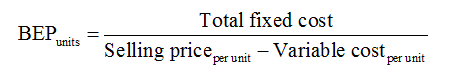# The following estimates have been prepared for a project: Fixed costs: \$36,000Depreciation: \$24,000Sales price per unit: \$4Accounting break-even: 40,000 units What must be the variable cost per unit? (Round your answer to 2 decimal places.)

Question
4 views

The following estimates have been prepared for a project:

Fixed costs: \$36,000
Depreciation: \$24,000
Sales price per unit: \$4
Accounting break-even: 40,000 units

What must be the variable cost per unit? (Round your answer to 2 decimal places.)

check_circle

Step 1

Variable cost is the cost which changes with the change in the output produced. In other words, the expenses incurred to produce the specified number of goods and services.

Step 2

Given Information:

Fixed costs are \$36,000

Breakeven point (in units) is 40,000

Depreciation expense is \$24,000

Sales price per unit is \$4

Step 3

Formula used:...

### Want to see the full answer?

See Solution

#### Want to see this answer and more?

Solutions are written by subject experts who are available 24/7. Questions are typically answered within 1 hour.*

See Solution
*Response times may vary by subject and question.
Tagged in